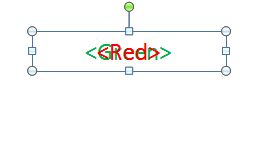# Qlik NPrinting Discussions

Discussion Board for collaboration on Qlik NPrinting.

Announcements
Coming your way, the Qlik Data Revolution Virtual Summit. October 27-29. REGISTER
cancel
Showing results for
Did you mean:
HighlightedCreator

## Text color in NPrinting power point

Hi Qlikies,

Is there a way we can do conditional color for text in the power point slide. Actually i am bringing qlik sense app KPI object expression as formula in Nprinting. And needs to show the color like >= 100 is green, < 100 is blue.

Thanks!

Pooja
Labels (1)
• ### Qlik NPrinting April 2019

1 Solution

Accepted Solutions
HighlightedMVP

Conditional formatting in PowerPoint does not exist and this is not really NPrinting question as it fits more in MsOffice community question...

but...

If you are talking just about KPI and want to do this directly in NPrinting the workaround would be:

• create PPT template
• for 1 KPI create 2 formulas with thresholds you need; for example:
• If(Sum(Expression1)>100,' ',Sum(Expression1)) - this would be formula calculating and returning value when greater than 100
• If(Sum(Expression1)<=100,' ',Sum(Expression1)) - this would be formula calculating and returning value when lesser than 100
• in PPT template you bring first formula and set it up and hard-code colour of it to red and you also put in the same position second formula and hardcode it to Green

Effectively you place both formula placeholders in the same spot in your template (one on top of another). If the formula does not meet "if condition" it will return nothing (be transparent) and for condition met it will return value in already hard-coded colour.

I understand this is lot of work, but for PPT that is the best i could come up with (except using images)

Check images for reference:

create formulas:drag formulas to the templateput formulas in exactly the same spot (one on top of another)cheers Lech, When applicable please mark the correct/appropriate replies as "solution" (you can mark up to 3 "solutions". Please LIKE threads if the provided solution is helpful to the problem.
2 Replies
HighlightedMVP

Conditional formatting in PowerPoint does not exist and this is not really NPrinting question as it fits more in MsOffice community question...

but...

If you are talking just about KPI and want to do this directly in NPrinting the workaround would be:

• create PPT template
• for 1 KPI create 2 formulas with thresholds you need; for example:
• If(Sum(Expression1)>100,' ',Sum(Expression1)) - this would be formula calculating and returning value when greater than 100
• If(Sum(Expression1)<=100,' ',Sum(Expression1)) - this would be formula calculating and returning value when lesser than 100
• in PPT template you bring first formula and set it up and hard-code colour of it to red and you also put in the same position second formula and hardcode it to Green

Effectively you place both formula placeholders in the same spot in your template (one on top of another). If the formula does not meet "if condition" it will return nothing (be transparent) and for condition met it will return value in already hard-coded colour.

I understand this is lot of work, but for PPT that is the best i could come up with (except using images)

Check images for reference:

create formulas:drag formulas to the templateput formulas in exactly the same spot (one on top of another)cheers Lech, When applicable please mark the correct/appropriate replies as "solution" (you can mark up to 3 "solutions". Please LIKE threads if the provided solution is helpful to the problem.
HighlightedCreator

Hi Lech,

Awesome!! Thank you for your suggestion. Sounds like a way.

Kudos to you.:)

Thanks!
Pooja